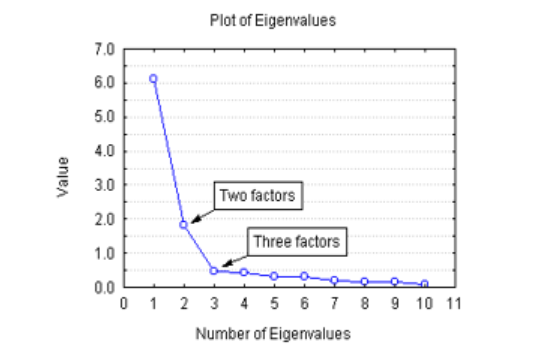# PCA (Principal Component Analysis)

dougieduk·2022년 4월 5일
0

# Concept

• reduce the number of variables of a data set while preserving as much information as possible
• a dimensionality-reduction method which transforms a large set of variables into a smaller one that still contains most of the information in the large set
• this comes at the expense of accuracy but the trick is to trade a little accuracy for simplicity

# Process

### compute the covariance matrix

• What is Covariance Matrix?
• p x p symmetric matrix that has the covariances associated with all possible pairs of initial variables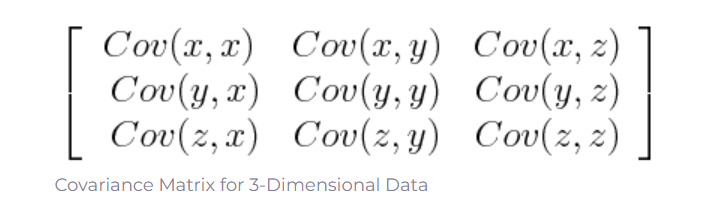• How do you compute it?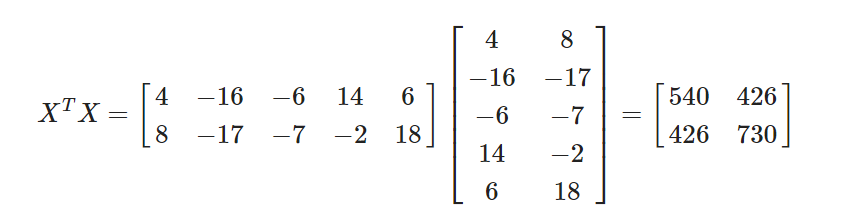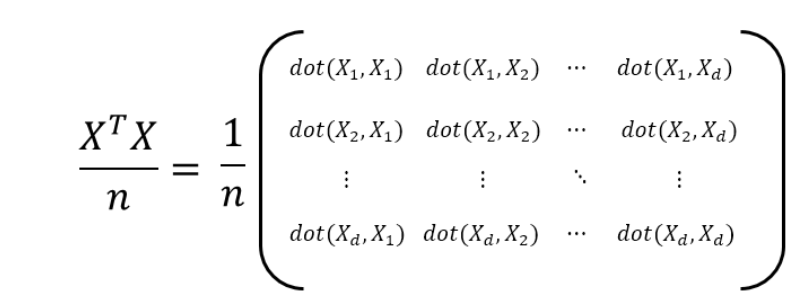### Compute the eigen vector and eigen value of the covariance matrix

• Definition of eigen vectors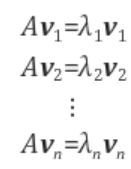• Determinant of (A-λE)has to be zero because otherwise, eigen vector will be zero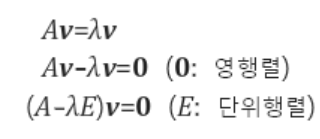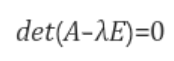Eigen Value is the variance of the model ( how much of the variables can be explained)

### How Many Dimensions are we going to use?

#### 1. Set a target variance

• e.g. 90% → sum of eigen value should be equal to this amount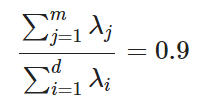#### 2. Use the elbow method

•